# How many valence electrons does carbon have?

Many of you have this question of how many electrons are in the last cell of carbon or how many valence electrons does carbon have?

In simple words, the number of electrons present in the valence shell (last electronic shell) is called valence electron.

The above two questions mean the same thing, so the carbon has four valence electrons. That is, there are 4 electrons present in the last cell of carbon.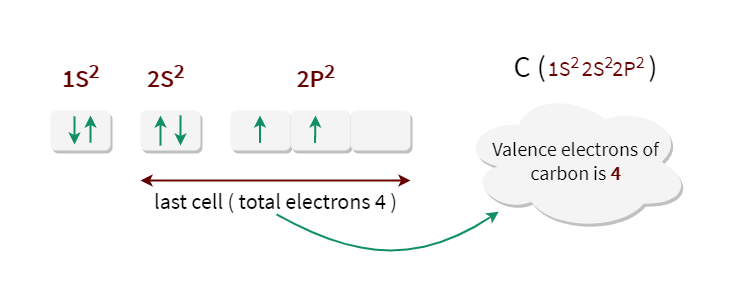## Electronic Configuration of Carbon

If you look at the electronic configuration of carbon, you can see that there are two cells present in carbon. The first cell has two electrons and the last cell has four electrons. So look at this illustration of the electric configuration of carbon.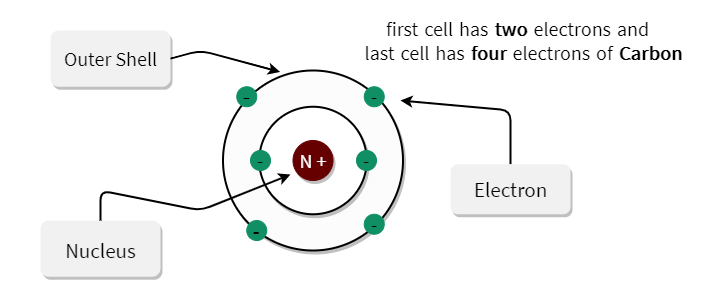Also, many of you are confused between the valency of carbon and valence electron. So, for this, you need to understand the difference between valency and valence electrons.

## What is Valancy of Carbon

According to the octet theory, in order for each element to reach a stable state, the number of electrons it leaves, or gain or mutual shares in order to fill its octet, we will call that element the valence. Thus, the valency of carbon is four.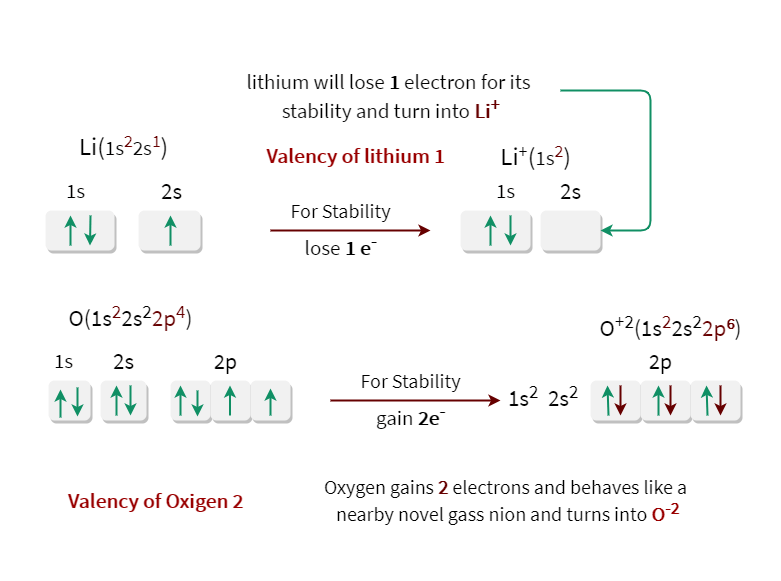After each element gains stability, its electronic configuration will be like the nearest Nobel gas.

Many of you may be confused as to where the word mutual share in definition came from.

There are some elements that can neither gain nor lose electrons in their last cell. In order for this element to be stable, the electrons in its last cell have to be mutually shared with other elements.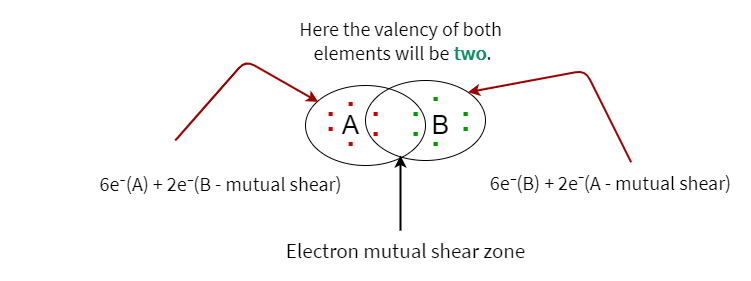An example is a carbon. Because it never becomes a c-4 or c+4 ion by accepting or rejecting the 4 electrons in the last cell in order to gain its stability. Because in this case, you have to give a lot of energy. For this, carbon always forms covalent bonds by mutually sharing its own 4 electrons.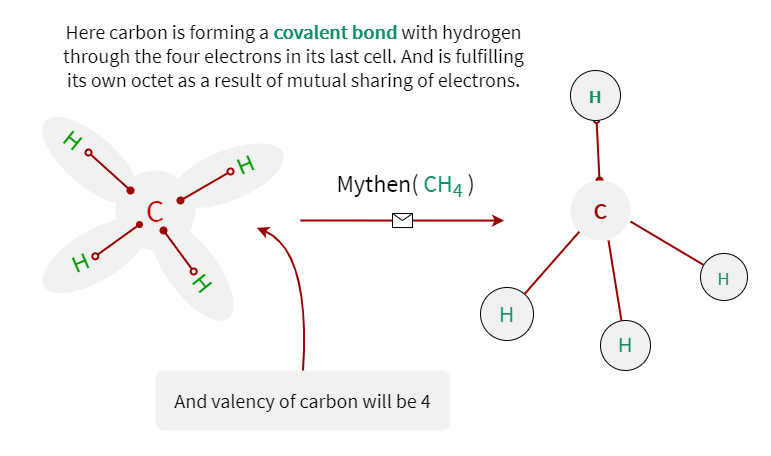## Question Answer on valency and valence electrons

The question-and-answer on valence and valence electrons is fully discussed below.

Problem1 In the case of carbon, valency, and valence electrons are equal. So will valence and valence electrons be equal or different in case of all other elements?

Valence electrons have no relation with Valency. Both are different concepts. Will be equal in the case of those elements whose number of electrons in the last cell will be equal to the number of electrons received or rejected for the stability of that element. Such as carbon, hydrogen, etc.

Problem2 What is the electron valency of neon and how many electrons are present in its last cell?

Neon is an inert element. That is, the neon will not receive or reject any electrons. In this case, the valency of neon will be zero. And the last cell of neon will always have 8 electrons. Thus, octet will remain met. The valency electron of neon will be 8.

Problem3 What is the valency electron of oxide ion?

If you look at the electronic configuration of oxide ions, you can see that there are 8 electrons present in the valence cell. Thus, the valency electron of oxide ion is 8.

Problem4 Give examples of elements other than carbon whose valency and valence electrons will be equal.

The valence of the hydrogen and the valence electron will both be equal. Hydrogen’s valence electron will be 1.

Problem5 Give examples of elements that have more than one valence.

Such as iron. It has two valences, two and three. Iron will lose two electrons at some point and turn into rt and at another time it will lose three electrons and turn into py. There are also many elements that have more than one valence.

Problem6 How many valence electrons does oxygen have?

The valence electron of oxygen will be 6. Because when you look at the electronic configuration of oxygen, there are 6 electrons present in the last cell(valence cell).

Problem7 How many valence electrons do Fluorine have?

The valence electron of fluorine is 7. That is, fluorine will gain 1 electron and gain the electronic configuration of neon. And the valency of fluorine will be 1.

Problem8 How many valence electrons does Nitrogen have?

There are five electrons present in the last cell of nitrogen. Thus, the valence electron of nitrogen will be five.

Problem9 How many valence electrons do Sodium have?

The valence electron of sodium will be one. Because the last cell of sodium i.e. 3s has only one electron.

## Conclusion

This tutorial discusses how many valence electrons there are in carbon. And also the valency of carbon is discussed in depth here. Here are some beautiful photos that have been added to the tutorial to make you understand.